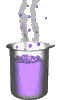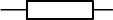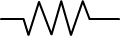Science class online
Chemistry and physics lessons

# Electricity

Free online electricity lessons for elementary school,  middle school and high school.Science class Chemistry Electricity Optics Mechanics Electricity lessons Electrical components -Two-terminal electronic components - Basic electrical components - Diodes - What's a resistor ? - How to determine a resistor value ? - Resistors effects in circuits - How to use a resistor ? - Characteristic curve of a resistor How to build simple circuits and draw diagrams - How to build a basic electrical circuit - How to draw diagrams of electric circuits The electric current - Conductors and insulators - Direction of electric current in a circuit - The dangers of electricity - Current intensity - units how to measure current ? The Voltage - The voltage and its units - How to measure a voltage ? - Voltage in open and closed circuits - Rated current and voltage for a lamp Alternating voltage and current - Alternating currents effects on led - What are alternating current and voltage ? - Periodic alternating voltage and its properties - Oscilloscope - Oscillogram - Frequency - Measuring RMS voltage with a voltmeter Serie circuits - What's a serie circuit ? - Series circuit properties - Short circuit in  series circuit - Current law in series - circuits - Voltage law in series circuits Parallel circuits - What's a parallel circuit ? - Some parallel circuits properties - Short circuits in parallel circuits - Nodes and branches in parallel circuits - Current laws in parallel circuits - Voltage laws in parallel circuits Laws of electricity - Ohm's law - Current laws in series circuits - Voltage laws in series circuits - Current laws in parallel circuits - Voltage laws in parallel circuits Generating electricity - Voltage for coil wires What is an alternator ? - Alternating currents and voltages - Generating electricity in power plants Electric power and energy - Electric power and power rating - Electric power consumption by an electrical device - Relationship between - Electric power and energy

 _____________________________________________________   _____________________________________________________

## Electrical components

### What is a resistor ?

A resistor is a cylindrical shape non-polarized component whose two terminals are identical.
Standard symbol of resistance:American standard symbol:Each resistor is characterized by a physical quantity called resistance
 This quantity is denoted by R and its unit is the ohm of symbol: Ω (Omega)
Example:
For a resistor with a value of 400 ohms we may note its value
R = 400 Ω
Comment:
Resistance not only characterizes the resistors but also other electrical components and conducting materials.
______________________________________

______________________________________

____________________________________

____________________________________